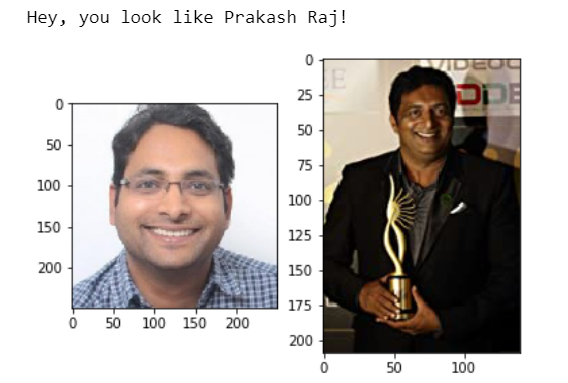# Celebrity Look-alike with Computer Vision - Find the Matching Celebrity Image

Finally, in this step we will find the matching celebrity image against the image we uploaded from the local computer. This is what the final output will look like:INSTRUCTIONS
1. Use the `calculate_face_distance()` function to calculate the Euclidean distance between the faces:

``````matching_image = << your code goes here >>(known_encodings, original_image)
``````
2. Now, let us check which celebrity do you look like:

``````import matplotlib.pyplot as plt
import matplotlib.image as mpimg
from matplotlib import rcParams

%matplotlib inline

# display images
fig, ax = plt.subplots(1,2)
ax.imshow(img_1);
ax.imshow(img_2);

print('Hey, you look like ' + os.path.splitext(matching_image) + '!')
``````